On Twisted Space

Delso J

Bachelor’s Degree in Physics by Zaragoza University, Spain

Article
Article Info
Author Info
Figures & Data

Abstract

In a manifold with a linear connection if the torsion tensor vanishes then the Christoffel symbols are symmetric in the lower indices, this is the motivation to call it the symmetric connection.

The requirement of being metric, the length is preserved by parallel transport and symmetric leads to the unique result for the Christoffel symbols of the connection.

This distinguished linear connection on a Riemannian manifold is usually called the Riemann connection or the Levi-Civita connection.

We consider a connection which is metric but not symmetric, the torsion tensor does not vanish and we study the conservation laws depending on it, the non-symmetric Ricci tensor and the action.

Key Words

Twisted Space; Torsion Tensor; Conservation Law; Curved Space; General Relativity; Riemann Curvature Tensor; Ricci Tensor

Introduction

Galaxies in our universe are rotating with such speed that the gravity generated by their observable matter could not possibly hold them together; an extra force is needed to explain it.

In a galaxy there are no dark matter particles generating the dark matter force to explain this extra force, new terms in the action depending on the torsion explain this extra force.

This new force also defines the distribution of galaxies in the universe.

A metric connection not symmetric

The Christoffel symbols of the first kind of the connection with a given torsion T satisfy .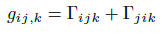(1)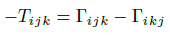(2)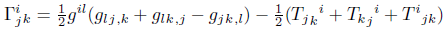(3)

Differentiating the determinant g  and defining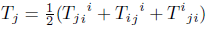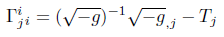(4)

The vector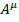has the covariant divergence .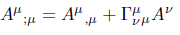(5)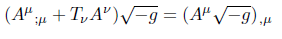(6)

If the left-hand side of equation (6) equals zero then the right-hand side gives us the first conservation law.

For the antisymmetric tensor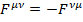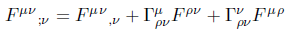(7)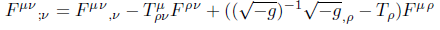(8)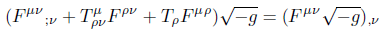(9)

If the left-hand side of equation (9) equals zero then the right-hand side gives us the second conservation law .

In the symmetric case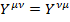we can get a corresponding equation, provided we put one of the suffixes downstairs and deal with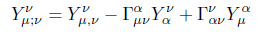(10)(11)

Since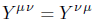is symmetric, we can replace the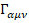from (1) by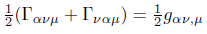(12)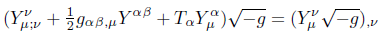(13)

For the antisymmetric tensor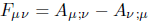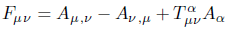(14)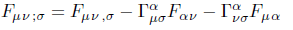(15)(16)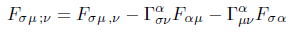(17)

Adding equations (15), (16) and (17)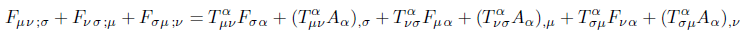(18)

From the definition of the curvature tensor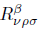.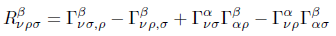(19)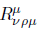is called the Ricci tensor .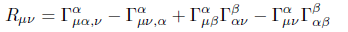(20)

Now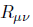is not symmetric,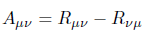is the antisymmetric part andwhere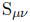is the symmetric part in the Einstein's equation.(21)

The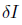for the antisymmetric tensor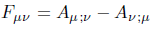.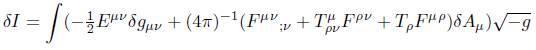(22)

whereis the stress-energy tensor of the field .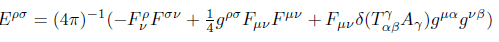(23)

The term,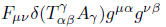, is derived from the new dependence of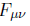on torsion tensor and the dependence of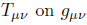.

The other new terms depending on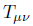are derived from equation (9).

Conclusions

Tensor densities have been defined leading to conservation laws depending on the torsion, if torsion is zero these equations return to the known conservation laws in General Relativity.

The antisymmetric part of the Ricci tensor has been defined depending on the torsion tensor; if torsion tensor vanishes the Ricci tensor is symmetric and leads to Einstein's equation. Action has been defined and there are new terms depending on the torsion, if the torsion tensor vanishes this equation return to the known action.

Space must also be twisted, for a complete image of the universe we need the antisymmetric part of the connection.

References

1. Marian Fecko (2006) Differential geometry and Lie groups for Physicists Cambridge University Press. Cambridge 389.
2. P.A.M. Dirac (1996) General Theory of Relativity. Princeton University Press 37.
3. P.A.M. Dirac (1996) General Theory of Relativity. Princeton University Press 38.
4. P.A.M. Dirac (1996) General Theory of Relativity. Princeton University Press 39.
5. P.A.M. Dirac (1996) General Theory of Relativity. Princeton University Press 21.
6. P.A.M. Dirac (1996) General Theory of Relativity. Princeton University Press 25.
7. P.A.M. Dirac (1996) General Theory of Relativity. Princeton University Press 54-55.

Article Type

Short Communication

Publication history

Accepted date: November 13, 2021
Published date: November 25, 2021

©2021 Delso J. This is an open-access article distributed under the terms of the Creative Commons Attribution License, which permits unrestricted use, distribution, and reproduction in any medium, provided the original author and source are credited.

Citation

Delso J. (2021) On Twisted Space. OSP Journal of Physics and Astronomy 2: JPA-2-123

Jesus Delso Lapuerta

Bachelor's Degree in Physics by Zaragoza University, Spain jesus.delso@gmail.com

No Figures